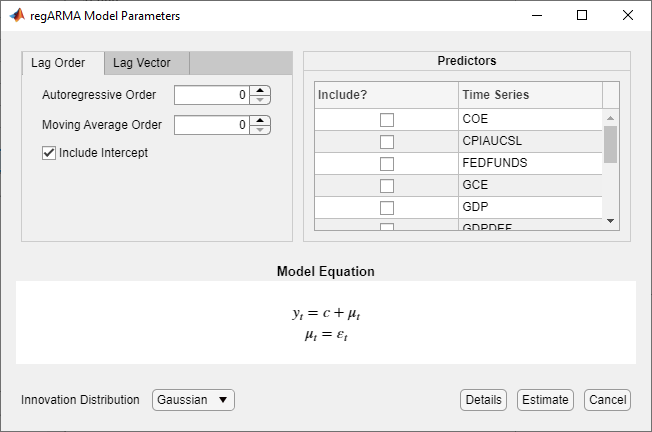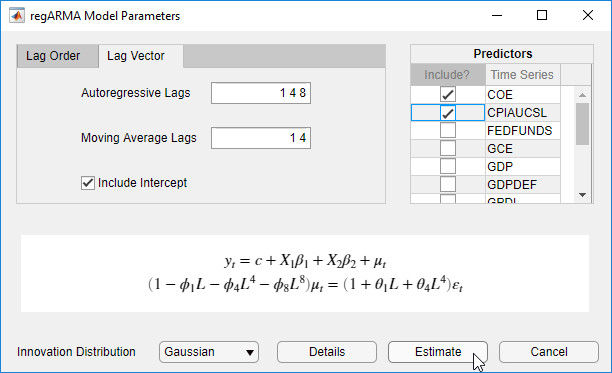Documentation

## Create Regression Models with ARMA Errors

### Default Regression Model with ARMA Errors

This example shows how to apply the shorthand `regARIMA(p,D,q)` syntax to specify the regression model with ARMA errors.

Specify the default regression model with ARMA(3,2) errors:

`$\begin{array}{l}{y}_{t}=c+{X}_{t}\beta +{u}_{t}\\ {u}_{t}={a}_{1}{u}_{t-1}+{a}_{2}{u}_{t-2}+{a}_{3}{u}_{t-3}+{\epsilon }_{t}+{b}_{1}{\epsilon }_{t-1}+{b}_{2}{\epsilon }_{t-2}.\end{array}$`

`Mdl = regARIMA(3,0,2)`
```Mdl = regARIMA with properties: Description: "ARMA(3,2) Error Model (Gaussian Distribution)" Distribution: Name = "Gaussian" Intercept: NaN Beta: [1×0] P: 3 Q: 2 AR: {NaN NaN NaN} at lags [1 2 3] SAR: {} MA: {NaN NaN} at lags [1 2] SMA: {} Variance: NaN ```

The software sets each parameter to `NaN`, and the innovation distribution to `Gaussian`. The AR coefficients are at lags 1 through 3, and the MA coefficients are at lags 1 and 2.

Pass `Mdl` into `estimate` with data to estimate the parameters set to `NaN`. The `regARIMA` model sets `Beta` to `[]` and does not display it. If you pass a matrix of predictors (${X}_{t}$) into `estimate`, then `estimate` estimates `Beta`. The `estimate` function infers the number of regression coefficients in `Beta` from the number of columns in ${X}_{t}$.

Tasks such as simulation and forecasting using `simulate` and `forecast` do not accept models with at least one `NaN` for a parameter value. Use dot notation to modify parameter values.

### ARMA Error Model Without an Intercept

This example shows how to specify a regression model with ARMA errors without a regression intercept.

Specify the default regression model with ARMA(3,2) errors:

`$\begin{array}{l}{y}_{t}={X}_{t}\beta +{u}_{t}\\ {u}_{t}={a}_{1}{u}_{t-1}+{a}_{2}{u}_{t-2}+{a}_{3}{u}_{t-3}+{\epsilon }_{t}+{b}_{1}{\epsilon }_{t-1}+{b}_{2}{\epsilon }_{t-2}.\end{array}$`

`Mdl = regARIMA('ARLags',1:3,'MALags',1:2,'Intercept',0)`
```Mdl = regARIMA with properties: Description: "ARMA(3,2) Error Model (Gaussian Distribution)" Distribution: Name = "Gaussian" Intercept: 0 Beta: [1×0] P: 3 Q: 2 AR: {NaN NaN NaN} at lags [1 2 3] SAR: {} MA: {NaN NaN} at lags [1 2] SMA: {} Variance: NaN ```

The software sets `Intercept` to 0, but all other parameters in `Mdl` are `NaN` values by default.

Since `Intercept` is not a `NaN`, it is an equality constraint during estimation. In other words, if you pass `Mdl` and data into `estimate`, then `estimate` sets `Intercept` to 0 during estimation.

You can modify the properties of `Mdl` using dot notation.

### ARMA Error Model with Nonconsecutive Lags

This example shows how to specify a regression model with ARMA errors, where the nonzero ARMA terms are at nonconsecutive lags.

Specify the regression model with ARMA(8,4) errors:

`$\begin{array}{l}{y}_{t}=c+{X}_{t}\beta +{u}_{t}\\ {u}_{t}={a}_{1}{u}_{1}+{a}_{4}{u}_{4}+{a}_{8}{u}_{8}+{\epsilon }_{t}+{b}_{1}{\epsilon }_{t-1}+{b}_{4}{\epsilon }_{t-4}.\end{array}$`

`Mdl = regARIMA('ARLags',[1,4,8],'MALags',[1,4])`
```Mdl = regARIMA with properties: Description: "ARMA(8,4) Error Model (Gaussian Distribution)" Distribution: Name = "Gaussian" Intercept: NaN Beta: [1×0] P: 8 Q: 4 AR: {NaN NaN NaN} at lags [1 4 8] SAR: {} MA: {NaN NaN} at lags [1 4] SMA: {} Variance: NaN ```

The AR coefficients are at lags 1, 4, and 8, and the MA coefficients are at lags 1 and 4. The software sets the interim lags to 0.

Pass `Mdl` and data into `estimate`. The software estimates all parameters that have the value `NaN`. Then `estimate` holds all interim lag coefficients to 0 during estimation.

### Known Parameter Values for a Regression Model with ARMA Errors

This example shows how to specify values for all parameters of a regression model with ARMA errors.

Specify the regression model with ARMA(3,2) errors:

`$\begin{array}{l}{y}_{t}={X}_{t}\left[\begin{array}{l}2.5\\ -0.6\end{array}\right]+{u}_{t}\\ {u}_{t}=0.7{u}_{t-1}-0.3{u}_{t-2}+0.1{u}_{t-3}+{\epsilon }_{t}+0.5{\epsilon }_{t-1}+0.2{\epsilon }_{t-2},\end{array}$`

where ${\epsilon }_{t}$ is Gaussian with unit variance.

```Mdl = regARIMA('Intercept',0,'Beta',[2.5; -0.6],... 'AR',{0.7, -0.3, 0.1},'MA',{0.5, 0.2},'Variance',1)```
```Mdl = regARIMA with properties: Description: "Regression with ARMA(3,2) Error Model (Gaussian Distribution)" Distribution: Name = "Gaussian" Intercept: 0 Beta: [2.5 -0.6] P: 3 Q: 2 AR: {0.7 -0.3 0.1} at lags [1 2 3] SAR: {} MA: {0.5 0.2} at lags [1 2] SMA: {} Variance: 1 ```

The parameters in `Mdl` do not contain `NaN` values, and therefore there is no need to estimate `Mdl` using `estimate`. However, you can simulate or forecast responses from `Mdl` using `simulate` or `forecast`.

### Regression Model with ARMA Errors and t Innovations

This example shows how to set the innovation distribution of a regression model with ARMA errors to a t distribution.

Specify the regression model with ARMA(3,2) errors:

`$\begin{array}{l}{y}_{t}={X}_{t}\left[\begin{array}{l}2.5\\ -0.6\end{array}\right]+{u}_{t}\\ {u}_{t}=0.7{u}_{t-1}-0.3{u}_{t-2}+0.1{u}_{t-3}+{\epsilon }_{t}+0.5{\epsilon }_{t-1}+0.2{\epsilon }_{t-2},\end{array}$`

where ${\epsilon }_{t}$ has a t distribution with the default degrees of freedom and unit variance.

```Mdl = regARIMA('Intercept',0,'Beta',[2.5; -0.6],... 'AR',{0.7, -0.3, 0.1},'MA',{0.5, 0.2},'Variance',1,... 'Distribution','t')```
```Mdl = regARIMA with properties: Description: "Regression with ARMA(3,2) Error Model (t Distribution)" Distribution: Name = "t", DoF = NaN Intercept: 0 Beta: [2.5 -0.6] P: 3 Q: 2 AR: {0.7 -0.3 0.1} at lags [1 2 3] SAR: {} MA: {0.5 0.2} at lags [1 2] SMA: {} Variance: 1 ```

The default degrees of freedom is `NaN`. If you don't know the degrees of freedom, then you can estimate it by passing `Mdl` and the data to `estimate`.

Specify a ${t}_{5}$ distribution.

`Mdl.Distribution = struct('Name','t','DoF',5)`
```Mdl = regARIMA with properties: Description: "Regression with ARMA(3,2) Error Model (t Distribution)" Distribution: Name = "t", DoF = 5 Intercept: 0 Beta: [2.5 -0.6] P: 3 Q: 2 AR: {0.7 -0.3 0.1} at lags [1 2 3] SAR: {} MA: {0.5 0.2} at lags [1 2] SMA: {} Variance: 1 ```

You can simulate or forecast responses from `Mdl` using `simulate` or `forecast` because `Mdl` is completely specified.

In applications, such as simulation, the software normalizes the random t innovations. In other words, `Variance` overrides the theoretical variance of the t random variable (which is `DoF`/(`DoF` - 2)), but preserves the kurtosis of the distribution.

### Specify Regression Model with ARMA Errors Using Econometric Modeler App

In the Econometric Modeler app, you can specify the predictor variables in the regression component, and the error model lag structure and innovation distribution of a regression model with ARMA(p,q) errors, by following these steps. All specified coefficients are unknown but estimable parameters.

1. At the command line, open the Econometric Modeler app.

`econometricModeler`

Alternatively, open the app from the apps gallery (see Econometric Modeler).

2. In the Data Browser, select the response time series to which the model will be fit.

3. On the Econometric Modeler tab, in the Models section, click the arrow to display the models gallery.

4. In the models gallery, in the Regression Models section, click .

The RegARMA Model Parameters dialog box appears.5. Choose the error model lag structure. To specify a regression model with ARMA(p,q) errors that includes all AR lags from 1 through p and all MA lags from 1 through q, use the Lag Order tab. For the flexibility to specify the inclusion of particular lags, use the Lag Vector tab. For more details, see Specifying Lag Operator Polynomials Interactively. Regardless of the tab you use, you can verify the model form by inspecting the equation in the Model Equation section.

6. In the Predictors section, choose at least one predictor variable by selecting the Include? check box for the time series.

For example, suppose you are working with the `Data_USEconModel.mat` data set and its variables are listed in the Data Browser.

• To specify a regression model with AR(3) errors for the unemployment rate containing all consecutive AR lags from 1 through its order, Gaussian-distributed innovations, and the predictor variables COE, CPIAUCSL, FEDFUNDS, and GDP:

1. In the Data Browser, select the `UNRATE` time series.

2. On the Econometric Modeler tab, in the Models section, click the arrow to display the models gallery.

3. In the models gallery, in the Regression Models section, click .

.

4. In the regARMA Model Parameters dialog box, on the Lag Order tab, set Autoregressive Order to `3`.

5. In the Predictors section, select the Include? check box for the COE, CPIAUCSL, FEDFUNDS, and GDP time series.

• To specify a regression model with MA(2) errors for the unemployment rate containing all MA lags from 1 through its order, Gaussian-distributed innovations, and the predictor variables COE and CPIAUCSL.

1. In the Data Browser, select the `UNRATE` time series.

2. On the Econometric Modeler tab, in the Models section, click the arrow to display the models gallery.

3. In the models gallery, in the Regression Models section, click .

4. In the regARMA Model Parameters dialog box, on the Lag Order tab, set Moving Average Order to `2`.

5. In the Predictors section, select the Include? check box for the COE and CPIAUCSL time series.

• To specify the regression model with ARMA(8,4) errors for the unemployment rate containing nonconsecutive lags

`$\begin{array}{c}{y}_{t}=c+{\beta }_{1}CO{E}_{t}+{\beta }_{2}CPIAUCS{L}_{t}+{u}_{t}\\ \left(1-{\alpha }_{1}L-{\alpha }_{4}{L}^{4}-{\alpha }_{8}{L}^{8}\right){u}_{t}=\left(1+{b}_{1}L+{b}_{4}{L}^{4}\right){\epsilon }_{t}\end{array},$`

where εt is a series of IID Gaussian innovations:

1. In the Data Browser, select the `UNRATE` time series.

2. On the Econometric Modeler tab, in the Models section, click the arrow to display the models gallery.

3. In the models gallery, in the Regression Models section, click .

4. In the regARMA Model Parameters dialog box, click the Lag Vector tab:

1. In the Autoregressive Lags box, type `1 4 8`.

2. In the Moving Average Lags box, type `1 4`.

5. In the Predictors section, select the Include? check box for the COE and CPIAUCSL time series.• To specify a regression model with ARMA(3,2) errors for the unemployment rate containing all consecutive AR and MA lags through their respective orders, the predictor variables COE and CPIAUCSL, and t-distributed innovations:

1. In the Data Browser, select the `UNRATE` time series.

2. On the Econometric Modeler tab, in the Models section, click the arrow to display the models gallery.

3. In the models gallery, in the Regression Models section, click .

4. In the regARMA Model Parameters dialog box, click the Lag Order tab:

1. Set Autoregressive Order to `3`.

2. Set Moving Average Order to `2`.

5. Click the button, then select `t`.

6. In the Predictors section, select the Include? check box for the COE and CPIAUCSL time series.

The degrees of freedom parameter of the t distribution is an unknown but estimable parameter.

After you specify a model, click to estimate all unknown parameters in the model.